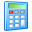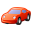Widgets# Drilling Horsepower Calculator

Calculate the horsepower required for a drilling operation based on the feed rate and tool diameter, which are used to determine the material removal rate (or metal removal rate). Also required is the unit power, which is a material property describing the amount of power required to cut that material. The horsepower at both the spindle and the motor are shown, as well as the spindle torque for a given spindle speed (RPM). The motor horsepower required for the drilling operation can then be compared to the horsepower capability of the machine. Learn more about Drilling.

## Drilling Horsepower Calculator

 Feed rate (IPM) × Tool diameter (in)² × (π/4)² × (π/4) = Material removal rate (in³/min)
 Material removal rate (in³/min) × Unit power (hp/in³/min) = Spindle horsepower (hp) Spindle horsepower (hp) ÷ Machine efficiency (%) / 100 / 100 = Motor horsepower (hp) Spindle horsepower (hp) × 63,030 × 63,030 ÷ Spindle speed (RPM) = Spindle torque (in∙lb)Get Widget!
Copy and paste the embed code below to include this widget on your webpage: